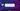# Max Priority Queue Implementation with Heap Data structure

July 10, 2019Max Priority Queue is a data structure which manage a list of keys(values). And, gives priority to element with maximum value.

It supports following operations:

1. getMax() - Gives the maximum priority element. Do not delete it.
2. extractMax() - Gives the maximum priority element, and delete it.
3. insert(element) - Insert an element into Priority queue
4. increment(index, newValue) - Increment an element from priority queue

(Heap data structure)[/coding-interview/what-is-heap-data-structure/] can be used for its implementation.

## Why not other data structure (other than Heap)?

Lets see each one of them:

• Link list Runtime complexity is O(n^2) in putting maximum element at top each time, a new element has come. Or, priority change for an element.
• Binary Search Tree Insertion is easy, getting maximum is an easy operation. It consumes extra space for keeping pointers for each node. In case of insertion and changing priority, tree needs to be re-balanced again, which is more complex than maintaining max heap property in heap data structure. Heap uses arrays, so accessing an element, caching an element are always faster operation.

## Implementation

``````public class MaxPriorityQueue {
private int[] heap;
private int maxSize;
private int heapSize;

public MaxPriorityQueue(int maxSize) {
this.maxSize = maxSize;
this.heap = new int[maxSize];
this.heapSize = 0;
}

@Override
public String toString() {
return ArrayUtils.toString(this.heap, this.heapSize);
}

private int getLeftChild(int index) { return 2*index + 1;}
private int getRightChild(int index) { return 2*index + 2;}
private int getParent(int index) {
if (index == 0) {
return -1;
}
return (index-1)/2;
}

private void maxHeapify(int index) {
int largest = index;
int l = this.getLeftChild(index);
int r = this.getRightChild(index);

if (l < this.heapSize && this.heap[l] > this.heap[index]) {
largest = l;
}
if (r < this.heapSize && this.heap[r] > this.heap[largest]) {
largest = r;
}
if (largest != index) {
ArrayUtils.swap(this.heap, largest, index);
this.maxHeapify(largest);
}
}

/**
* Get the max element, do not delete
*/
public int getMax() throws Exception {
if (this.heapSize == 0) {
throw new Exception("Heap underflow");
}
return this.heap;
}

/**
* Get the max, and delete from heap
* Runs in O(log n)
*/
public int extractMax() throws Exception {
if (this.heapSize == 0) {
throw new Exception("Heap underflow");
}

int ret = this.heap;
this.heap = this.heap[this.heapSize-1];

this.heapSize --;
this.maxHeapify(0);

return ret;
}

/**
* Set the value at index specified to new value specified
*/
public void increment(int index, int newValue) throws Exception {
if (index > this.heapSize-1) {
throw new Exception("Overflow");
}

this.heap[index] = newValue;
while (index > 0 && this.heap[index] > this.heap[this.getParent(index)]) {
ArrayUtils.swap(this.heap, index, this.getParent(index));
index = this.getParent(index);
}
}

public void insert(int val) throws Exception {
if (this.heapSize >= this.maxSize) {
throw new Exception("Overflow");
}
this.heapSize ++;
this.heap[this.heapSize-1] = Integer.MIN_VALUE;
this.increment(this.heapSize-1, val);
}

public static void main(String[] args) throws Exception {
MaxPriorityQueue q = new MaxPriorityQueue(10);

//fresh state
System.out.println(q);

//lets insert 5 elements
q.insert(10); q.insert(5); q.insert(4); q.insert(6); q.insert(20);
System.out.println(q);
System.out.println(q.getMax());

System.out.println("Extracting max: " + q.extractMax());
System.out.println("State: " + q);

System.out.println("Incrementing index-2 to 12");
q.increment(2, 12);
System.out.println("State: " + q);

System.out.println("Extracting max: " + q.extractMax());
System.out.println("State: " + q);
System.out.println("Extracting max: " + q.extractMax());
System.out.println("State: " + q);
}
}``````

## Explanation

To know about basic Heap data structure, and its heapify operation. See: (Heap data structure)[/coding-interview/what-is-heap-data-structure/] Its an important data structure for interview purpose.

### getMax()

Since we are maintaining max heap. The largest value is always at 0th index. Just return it. Runtime complexity is O(1)

### extractMax()

Runtime complexity is O(log n)

• Get the top element, 0th index. Which is max.
• For deletion, simply copy the last element to 0th index.
• Decrease heap size by 1. Means, the last element index which we copied is out of heap scope now.
• Now, since we have copied the last element to top. We have to maintain our Max-Heap property.
• Call maxHeapify()

### increment(index, newVal)

Runtime complexity is O(log n)

• Simply, set heap value at specified index to new value.
• Now, since that element was already larger than all of its child. The newer value is even greater then current value. So, the tree below this node is all max-heapified.
• We need to maintain max-heapify property in upper tree.
• Go till the root element, and swap this node value with its parent untill it is greater than its parent.
• So, its kind of bubbling up.

### insert(element)

Runtime complexity is O(log n)

• Increase heapsize for this new element
• Put a minimum value(minimum value of an integer) at the newer index (last index)
• Simply call increment() method for this index, and the value we want to insert.